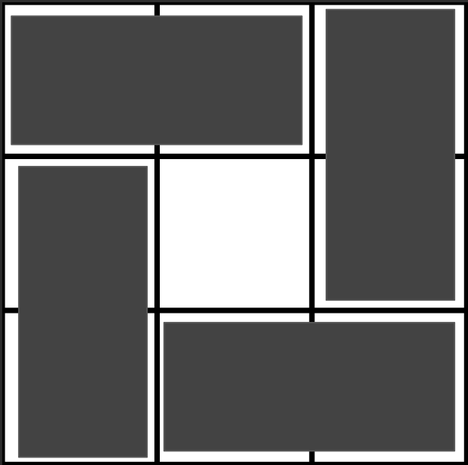# All In One GridAdilOlinBattle of Brains 2023, Un...14/17/20
Limits 1s, 512 MB

Ryana, Fardin, and Bithi are best friends. One day, they were playing with dominos and a grid. Ryana had a collection of $1 \times 2$ dominos, and Fardin had a grid of size $n\times m$. Bithi, being the puzzle master, challenged them to find the maximum number of dominos that can be used to tile the grid, following specific rules:

• You can place a domino in the grid either vertically $(2 \times 1)$ or horizontally $(1 \times 2)$.

• No two dominos can overlap; they must be placed in distinct grid positions.

• Every domino must be entirely inside the grid.

Ryana and Fardin, being clever, quickly solved the problem. Now, it's your turn to find the maximum number of dominos that can be tiled into the grid following Bithi's rules.

## Input

The first line contains $t$ $(1\leq t \leq 10)$ describing the number of test cases. This is followed by the description of each test case.

Next $t$ line contains two positive integers $n$ and $m$ describing the size of the grid for each test case. It is guaranteed that $n \times m \leq 100$.

## Output

For each test case, print a single line containing the maximum number of dominos that can be placed on the given grid by following the rules.

## Sample

InputOutput
2
3 3
1 2

4
1


A possible construction for the first test case,A possible construction for the second test case,You can verify that this is indeed the maximum number of dominos that can be used.

### Submit